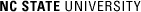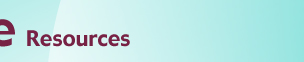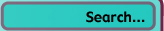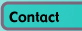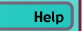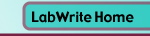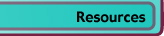# Graphing with Excel

## Basic Data Input in Excel

### Introduction

Excel is a type of computer program called a spreadsheet. It is probably the most widely used program for the management and analysis of numeric data. The utility of Excel and other spreadsheet programs comes from its visual method of storing and management of data. Each unique bit of data is held in a cell. Each cell has a unique location and can be referenced to format the display of the numeric data, create graphs, and perform mathematical operations on the data.

### Inputting data

You can enter data by simply clicking on one of the cells in the spreadsheet and typing in your values. Normally you will start entering somewhere near the upper left corner of the spreadsheet. You can move to the next cell in a number of ways:

• Clicking on the next cell you want to enter data into
• Return will move to the next cell down
• Tab will move to the next cell to the right
• Arrow keys will move in the direction of the arrow

Referring to the figure below, the first row of data might be entered:

1 Tab 52 Tab 48 Tab 73.5

The first column might be entered:

1 Return 3 Return 2 Return 5 Return 2

Data in a cell can be deleted by highlighting a cell and either typing in a new value or hitting Delete.

### Identifying cells

Each cell is located in a rectilinear grid of cells and is located by a column and row designation. Columns are designated by letters while rows are designated by numbers.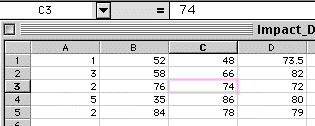In this piece of an Excel spreadsheet, the number 74 is located in cell C3. Notice that the location designation of the highlighted cell is shown on the right of the first tool bar above the spreadsheet (called the formula bar). Similarly, the value of the cell is also shown in the formula bar. Every other number in this spreadsheet can be located with a unique letter-number designation.

### Cell ranges

Groups of cells can also be specified by placing a colon between the upper left and lower right corners of the group of cells. For example: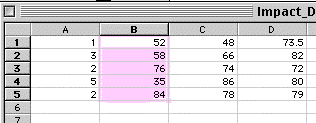This highlighted column of cells would be B1:B5. Notice that the letter designation is the same for a single column of cells. Another selection might be: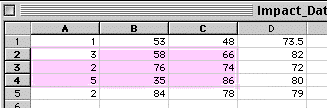Here, multiple rows and columns are selected and would be designated as A2:C4.

### Entering formulas

The power of spreadsheets is not just in being able to hold numbers, but also manipulating them with mathematical formulas. A cell is designated as having a formula in it by typing an equal (=) sign as the first character. Next comes a combination of cell designations and/or standard mathematical operators (+, -, *, /) and/or special predefined functions. For example: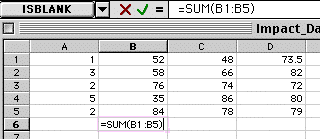A formula is entered in cell B6, starting with the equal sign followed by the special function SUM(). The cells to be summed are specified inside the parentheses. In this case cells B1 to B5 are summed. The entry of B1:B5 into the function could have been done by typing the letters or by clicking and dragging on the range of cells of interest. Note that the formula is also shown up in the formula bar. You can accept the formula either by typing return or by clicking on the green checkmark to the left of the formula. Cell B6 now shows the result of the formula calculation: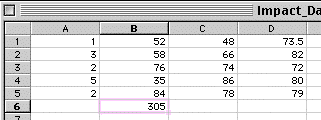A complete list of functions can be found by clicking on a cell and choosing Insert>Function...

### Relative versus absolute cell references

Most formulas are applied numerous times to different groups of cells, referring to a different range of cells each time it is used in a different cell location. Because of this, Excel provides a behavior to speed the updating of cell references. Supposed you had your SUM formula entered in B6 and you wanted to use it to sum the values in column C. You might select B6, choose Edit>Copy, select C7, and choose Edit>Paste: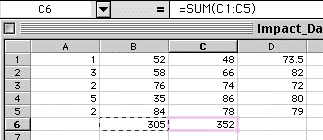Notice up in the formula bar that the SUM function now operates on C1:C5. When the formula was copied over one column (B6 to C6), the range of cells referred to in the function also shifted one column (B1:B5 to C1:C5). This is because the cell references in the function were relative references.

If you do not want the cell(s) that a formula refers to shift relative to where the formula is copied, you must use absolute references. You can do so by putting a dollar (\$) sign before the letter and number in the cell designation. For example, say your formula refers to a constant located in cell E7: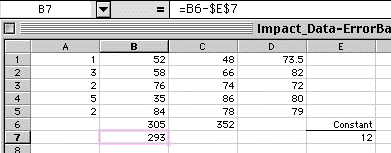If you had simply entered the cell reference as E7, when the formula in B7 was copied to C7, the reference to E7 would have shifted to F7. This would be undesirable. Instead the constant is referenced with \$E\$7. Now when it is copied: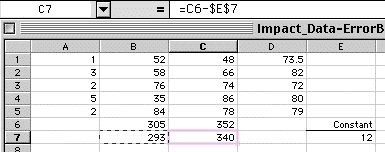The formula in C7 still refers to cell E7.

### Formatting cells

Often when data are inputted into cells, the numeric values are not formatted the way you would like them to appear in the spreadsheet or in graphs you will later create. The number of decimal places, along with many other formatting options can be set by selecting the cell or cells you would like to format and choosing Format>Cells...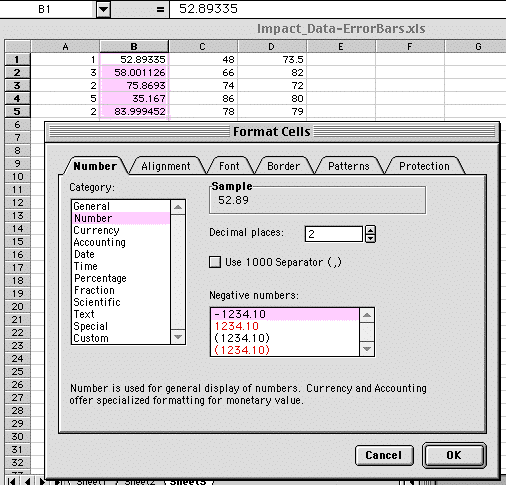When you first click on the Number tab, your cells will be listed in the Category General. This is the default data category for a new spreadsheet. To control the decimal places, you will need to change the Category to Number. Now type or click the arrows to set the appropriate number of Decimal places. A preview of what your formatted cell(s) will look like is shown in the Sample box. You can also control how Negative numbers appear. For scientific applications, you usually leave it at the default seen here.

Though in this example, the number of decimal places has been changed to two places, the underlying value stored in the spreadsheet has not been rounded: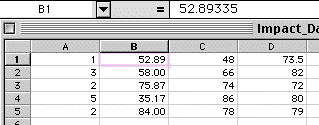Note for the highlighted cell, the full five decimal places still show up in the formula bar.

The number of decimal places can also be controlled with the tool bar icons: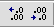© Copyright NC State University 2004 Sponsored and funded by National Science Foundation (DUE-9950405 and DUE-0231086) Site design by Rosa Wallace Rev. RW 5/16/05